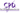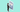Learn the basics of

# Data Analytics

If you want to become a data analyst pro, this course is for you. This course will help you analyse data to make better decisions and add a solid foundation in statistics to better interpret your data. Before taking this course, you should be familiar with organizing and summarizing data using Excel analytic tools, such as tables, pivot tables, and pivot charts. You should also be comfortable (or willing to try) creating complex formulas and visualizations. By the end of this course, the student will be able to conduct Data Analysis using 3 popular Data analysis tools- Excel, R and Tableau. The student will learn valuable core statistical skills that will solidify their understanding of data analysis. They will learn how to do basic data analysis in Excel, intermediate level analysis in R using various packages and build dashboards in Tableau.

The data set used in this Module can be found at the following link: www.kaggle.com/c/titanic/data. Download the dataset called "train.csv".

612K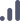All levels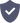Certified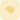# Course details

+

-

Your journey to becoming a data analyst will start by understanding more about data and the analysis thereof. This lesson is geared to help you understand why data analysis is an important skill as well as where it can be used to enhance your business making decisions. Each one of our lessons is carefully balanced between theory and practical and in this lesson, you can expect to learn how to import and clean data using a variety of methods and tools. We will focus on logical checks to help guide you towards thinking about data in a more logical fashion.

2.Exploring Data

+

-

In this lesson, we will understand the data in a bit more detail. The aim is to assist you in understanding the different data types (such as categorical vs numerical) as well as understanding graphically represented data. You will also learn how to describe data (i.e. descriptive statistics) and how to implement descriptive statistics "features" in data.

3.Probability

+

-

As our journey continues, you will learn how to install the Data Analysis Toolpak together with some descriptive stats. We will touch briefly on the basics of probability (with specific reference to Bayes theorem) and delve into the details of mean and variance of random variables. This topic very neatly ties together a concept that we have covered (the mean) with one we are yet to cover (variance).

4.Distributing Data

+

-

Lesson 4 is all about distributing data. You will learn about the various data distributions (with reference to the central limit theorem) and understand how to use mean, median and standard deviation to know how your data is distributed. Lastly, we will also expand on skewness and kurtosis, so tune in if you are dying to know what this means for your data.

5.How Confident Are You In The Sample?

+

-

Being confident in your sample is important. This lesson will focus on understanding the difference between a sample and a population as well as when to use variance or standard deviation for each. We will also cover confidence intervals in more detail, and by the end of this lesson you will be well on your way to feeling more confident!

+

-

Understanding what a hypothesis is an important step in your journey. We will expand on what a null and alternative hypothesis is and explore the difference between a Type 1 and Type 2 error. This lesson will also include more information on the central limit theorem/ the law of large numbers to enlighten us on this topic.

7.Testing For Differences: Categorical Vars

+

-

Our penultimate lesson is focused on testing for differences (categorical vars). We will explore one sample tests, the difference between 2 means of 2 populations as well as Chi-square tests.

8.Testing For Differences: Numerical Vars

+

-

Finally, we wrap up with understanding testing for differences (numerical vars). In this lesson one sample tests, the difference between 2 means of 2 populations and T-tests will be covered and by the end of this lesson you will have a firm and complete understanding of the basics of data and data analysis. The journey does not stop here and in Module 2 you can expect more complex concepts and a deeper understanding of the topic.

###### Certified by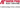###### Globally recognised by